﻿ Maths And Science Formula On Whiteboard Stock Photo - Image of male, algebra: 26095848

# Maths and science formula on whiteboard.

## Male teacher writing various high school maths and science formula on whiteboard

Designers also selected these stock photosCapsule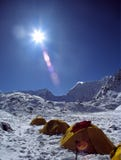Himalayan Base CampStock market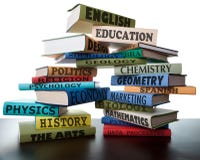Stack of school textbooks college study books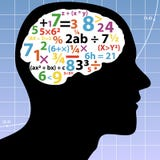Problem SolvingBackstabber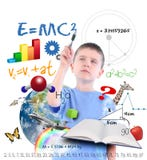Science Education School Boy Writing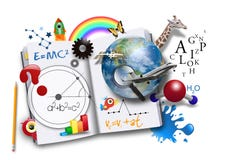Open Learning Book with Science and Math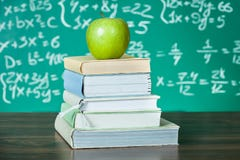Stack of school textbooks
More similar stock images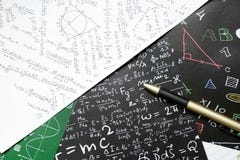Math problems on graph paper with pencil ,formula arithmetic using for concept of World Maths Day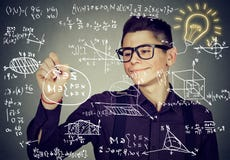Guy writing high school maths and science formulas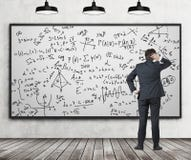Man in suit looking at whiteboard with formulas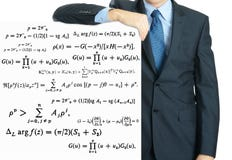male teacher writing various high school maths and science formula on whiteboard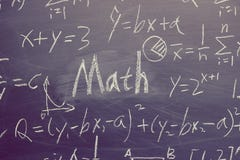Math Concept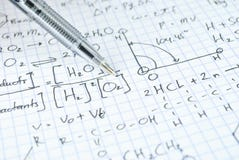Hand writing various high school maths and scienceComposite image of science graphicTeacher writes maths and science formula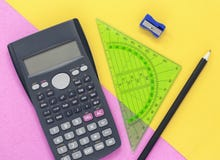Colourful pink and yellow desk flat lay with calculator
Related categories
Extended licenses